Courses

# JEE(MAIN) Mathematics Mock Test - 5

## 30 Questions MCQ Test JEE Main Mock Test Series 2020 & Previous Year Papers | JEE(MAIN) Mathematics Mock Test - 5

Description
This mock test of JEE(MAIN) Mathematics Mock Test - 5 for JEE helps you for every JEE entrance exam. This contains 30 Multiple Choice Questions for JEE JEE(MAIN) Mathematics Mock Test - 5 (mcq) to study with solutions a complete question bank. The solved questions answers in this JEE(MAIN) Mathematics Mock Test - 5 quiz give you a good mix of easy questions and tough questions. JEE students definitely take this JEE(MAIN) Mathematics Mock Test - 5 exercise for a better result in the exam. You can find other JEE(MAIN) Mathematics Mock Test - 5 extra questions, long questions & short questions for JEE on EduRev as well by searching above.
QUESTION: 1

Solution:
QUESTION: 2

Solution:
QUESTION: 3

### [(1+cosθ+i sinθ)/(i+sinθ+i cosθ)]4 = cos nθ + isin nθ, then n=

Solution:
QUESTION: 4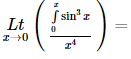Solution:
QUESTION: 5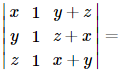Solution:
QUESTION: 6

The differential equation which represents the family of plane curves y=exp. (cx) is

Solution: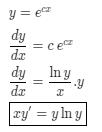QUESTION: 7

If the domain of function f(x) = x2 - 6x + 7 is (-∞, ∞), then the range of function is :

Solution:
QUESTION: 8

In the following question, a Statement-1 is given followed by a corresponding Statement-2 just below it. Read the statements carefully and mark the correct answer-
Let a, b, c, p, q be real numbers. Suppose α,β are the roots of the equation x2 + 2px + q = 0 and a, 1/β are the roots of the equation ax2 + 2bx + c = 0, where β2 ∉ {-1, 0, 1}
Statement-1:
(p2-q)(b2-ac)≥0
Statement-2:
b≠pa or c≠qa

Solution: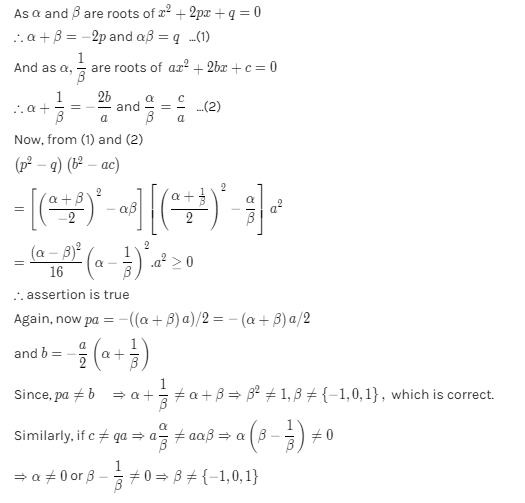QUESTION: 9

The angle between the pair of tangents drawn from the point (1,2) to the ellipse 3x2 + 2y2= 5 is

Solution:
QUESTION: 10

(d/dx)[cos(1-x2)2]=

Solution:
QUESTION: 11

In the following question, a Statement of Assertion (A) is given followed by a corresponding Reason (R) just below it. Read the Statements carefully and mark the correct answer-
Assertion(A): ex , loge x are two functions such that each is the image of the other with respect to the line x = y
Reason (R): The inverse of every bijective function is symmetric about the line x = y

Solution:
QUESTION: 12

A square tank of capacity 250 cubic m has to be dug out. The cost of land is Rs 50 per sq.m. The cost of digging increases with the depth and for the whole tank is 400 (depth)2 rupees. The dimensions of the tank for the least total cost are

Solution:
QUESTION: 13

The same roots of 3-4 i are

Solution:
QUESTION: 14

Everybody in a room shakes hands with every body else. The total number of hand shakes is 66. Then the number of persons in the room is

Solution:

There are n people in the room
n(n - 1)/2 = 66
n2 - n = 132
n2 - n - 132 = 0
n2 - 12n + 11n - 132 = 0
n(n - 12) + 11(n - 12) = 0
(n + 11) (n - 12) = 0
n = 12, - 11
Hence total no of persons = 12

QUESTION: 15

If the sum of the squares of the roots of x2 + px - 3 = 0 is 10, then the values of p =

Solution: Sum of the roots of equation :- α + β = - b/aProducts of the roots of equation :- αβ = c/a squaring on both side sum of roots of equation α^2 +β^2 +2αβ = b^2/a^2 put values in above equation 10 + 2(-3) =b^2 b = +2 or b = -2
QUESTION: 16

Three identical dice are rolled. The probability that the same number will appear on each of them, is

Solution:
QUESTION: 17
If the two lines of regression are 5x + 3y = 55 and 7x + y = 45, then the correlation coefficient between x and y is
Solution:
QUESTION: 18

If A is the single A.M. between two numbers a and b and S is the sum of n A.M.'s between them, then S/A depends upon

Solution:
QUESTION: 19

M telegrams are distributed at random over N communication channels (N > M). The probability of the event
A = {not more than one telegram will be sent over any channel} is

Solution:

The total no. of ways of distributing M telegrams over N channels = NM
The number of ways of choosing M channels out of N to send one telegram over each channel = NCM
∴ Total no. of ways to send M telegrams over each channel = NCM . M!
∴ Required probubility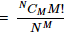QUESTION: 20

The equation of a line passing through (-a,0) and form a triangle of area 'T' with coordinates axes, is

Solution:
QUESTION: 21
The distance between the parallel planes x + 2y - 3z = 2 and 2x + 4y - 6z + 7 = 0 is
Solution:
QUESTION: 22

The co-ordinates of a point P are (3,12,4) w.r.t. the origin O, then the direction consines of OP are

Solution:
QUESTION: 23

sin 163° cos347° + sin 73° sin 167°

Solution:

sin 163° cos 347 + sin 73° sin 167
= sin (180° - 17°) cos (360° - 13°) + sin (90° - 17°) sin (180° - 13°)
= sin 17° cos 13° + cos 17° sin 13°
= sin (17° + 13°) = sin 30° = 1/2

QUESTION: 24

If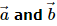represent the sides AB and BC of a regular hexagon ABCDEF, then the vector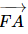equals

Solution:
QUESTION: 25

The integral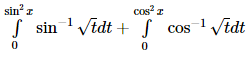is equal

Solution:
QUESTION: 26

A line has intercepts a, b on the coordinate axes. If the axes are rotated about the origin through an angle α then the line has intercepts p, q on the new position of the axes respectively. Then:

Solution:
QUESTION: 27

Let the equation of a curve be x = a (θ + sin θ), y = a(1 − cos θ). Let θ change at a constant rate k then the rate of change of the slope of the tangent to the curve at θ = π/3 is

Solution: Basically it is asking d2y/dx2 at (theta = π/3)

dy/dx = (dy/dz)/(dx/dz) where z is theta

dy/dz = asinz
dx/dz = a(1+cosz)
where z is theta

so dy/dx = sinz/(1+cosz)
=tan(z/2)

second differential = k× 1/2 {sec(z/2)}^2

at z=π/3

QUESTION: 28

In the following question, a Statement of Assertion (A) is given followed by a corresponding Reason (R) just below it. Read the Statements carefully and mark the correct answer-
Assertion(A): If y = 4x - 5 is a tangent to the curve y2 = px3 + q at (2,3) , then p = 2 and q = -7.

Reason: If tangent is parallel to x-axis, θ = 0°,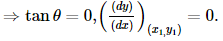Solution:
QUESTION: 29

In the following question, a Statement of Assertion (A) is given followed by a corresponding Reason (R) just below it. Read the Statements carefully and mark the correct answer-
Assertion(A): The chord of the hyperbola 25x2 - 16y2 = 400 whose middle point is (6,2) if 16x - 75y = 418.
Reason(R): Chord whose mid-point is (h,k) is given by T = S1.

Solution:
QUESTION: 30

In the following question, a Statement of Assertion (A) is given followed by a corresponding Reason (R) just below it. Read the Statements carefully and mark the correct answer-
Assertion(A): The values of p for which the equation 2x2 - √2px + p = 0 has equal roots are 0 and 4.
Reason(R): Equation ax2 + bx + c = 0 has real and equal roots, if its discriminant is zero.

Solution: